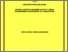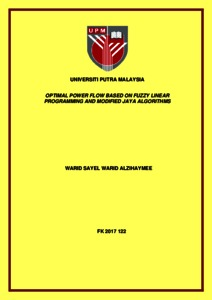# Optimal power flow based on fuzzy linear programming and modified Jaya algorithms

## Citation

Alzihaymee, Warid Sayel Warid (2017) Optimal power flow based on fuzzy linear programming and modified Jaya algorithms. Doctoral thesis, Universiti Putra Malaysia.

## Abstract

Optimal power flow (OPF) solution is a crucial tool in electric power networks operation. Recently, several factors, including the deregulated electricity markets, electricity consumption growth, the growing role of decision makers, and the increasing exploitation of distributed generation (DG), affect the operation strategies of many meshed power networks. These new conditions have raised the intricacy of OPF problems and necessitate a reliable optimization algorithm that can tackle economic and security concerns. A set of modified and novel optimization algorithms are proposed in this thesis to deal with different single and multi-objective OPF problems. A new formulation for the multi-objective optimal power flow (MOOPF) problem that considers DG is introduced. The proposed algorithms have been examined and validated using the IEEE 30-bus and IEEE 118-bus test systems. The first proposed approach is a multi-objective fuzzy linear programming optimization (MFLP) algorithm to solve the MOOPF problem. The results indicate that a unique and optimum solution with an excellent satisfaction for the extreme targets can be achieved. Secondly, the application and modification of a Jaya algorithm to deal with different OPF problems is presented. The main advantage of this algorithm is that no algorithm-particular controlling parameters are required for this algorithm. Two versions of the Jaya algorithm namely, the basic Jaya algorithm and novel quasi-oppositional Jaya (QOJaya) algorithm are proposed to solve different single objective OPF problems. In the proposed novel QOJaya algorithm, an intelligence strategy, namely, quasi-oppositional based learning (QOBL) is incorporated into the basic Jaya algorithm to enhance its convergence speed and solution optimality. For each considered case, results demonstrate that Jaya algorithm can produce a global optimum solution with rapid convergence. Meanwhile, the proposed QOJaya algorithm produces better results than the basic Jaya method in terms of solution optimality and convergence speed. In addition, two novel Jaya-based methods namely, the modified Jaya (MJaya) algorithm and quasi-oppositional modified Jaya (QOMJaya) algorithm are proposed to solve different MOOPF problems. In this work, a considerable contribution has been made in terms of modifying Jaya algorithm for handling MOOPF problems. Results show the applicability, potential, and efficacy of the proposed MJaya and QOMJaya algorithms in solving MOOPF problems. Finally, two novel hybrid optimization algorithms namely, FLP-QOJaya algorithm for single objective OPF problems and MFLP-QOMJaya algorithm for MOOPF problems are proposed. For all single objective OPF cases, results demonstrate that the FLP-QOJaya algorithm outperforms the proposed Jaya and QOJaya algorithms in terms of solution quality, convergence speed and execution time. For multi-objective OPF problems, results show the supremacy of the proposed MFLP-QOMJaya over the proposed MJaya and QOMJaya algorithms in terms of producing superior Pareto optimal solutions and finer best compromise solutions.Preview
Text
FK 2017 122 - IR.pdfView Item